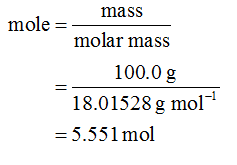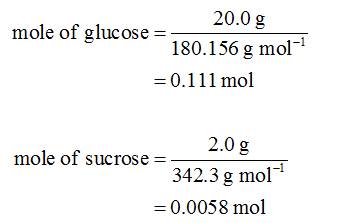# Which solution has a higher vapor pressure at 25 degress Celsius: 20.0g of glucose in 100.0mL of water OR 2.0g of sucrose in 100.0mL of water? Density of water is 1.00g/mL.

Question
1 views

Which solution has a higher vapor pressure at 25 degress Celsius: 20.0g of glucose in 100.0mL of water OR 2.0g of sucrose in 100.0mL of water? Density of water is 1.00g/mL.

check_circle

Step 1

Given information:

• 20.0g of glucose in 100.0mL of water
• 2.0g of sucrose in 100.0mL of water
• Density of water is 1.00g/mL
Step 2

The density of water is given as 1.00 g/mL. The mass of 100.0 mL of water is 100.0 g. The mole of water is calculated as follows:Step 3

The molar mass of glucose and sucrose is 180.156 g/mol and 342.3 g/mol respectively. The mole of glucose and sucrose is calculated as follows:...

### Want to see the full answer?

See Solution

#### Want to see this answer and more?

Solutions are written by subject experts who are available 24/7. Questions are typically answered within 1 hour.*

See Solution
*Response times may vary by subject and question.
Tagged in